# Foundations of convolutional neural networks - Class review

Last updated on：a year ago

Convolutional neural networks are widely used in computer vision because it can help you compress your network system.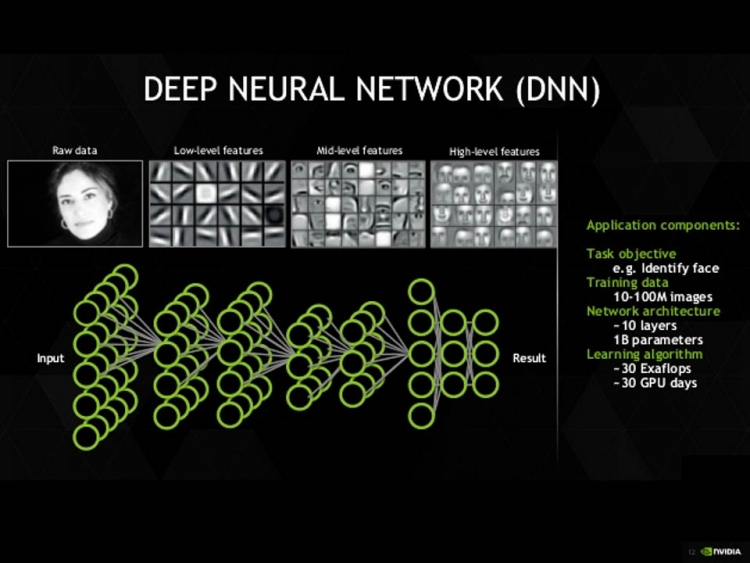# Computer Vision problems

CNNs (convolutional neural networks) allows abstracting an object’s identity or category from the specifics of the visual input (e.g., relative positions/orientation of the camera and the object).

• image classification

• object detection

• neural style transfer

Directly modifying the output part of residual block would cause lower performance of the system, because skip connection has a fully copied tensor which could be interrupted by the not proper output design.

# Edge detection example

Vertical edge detection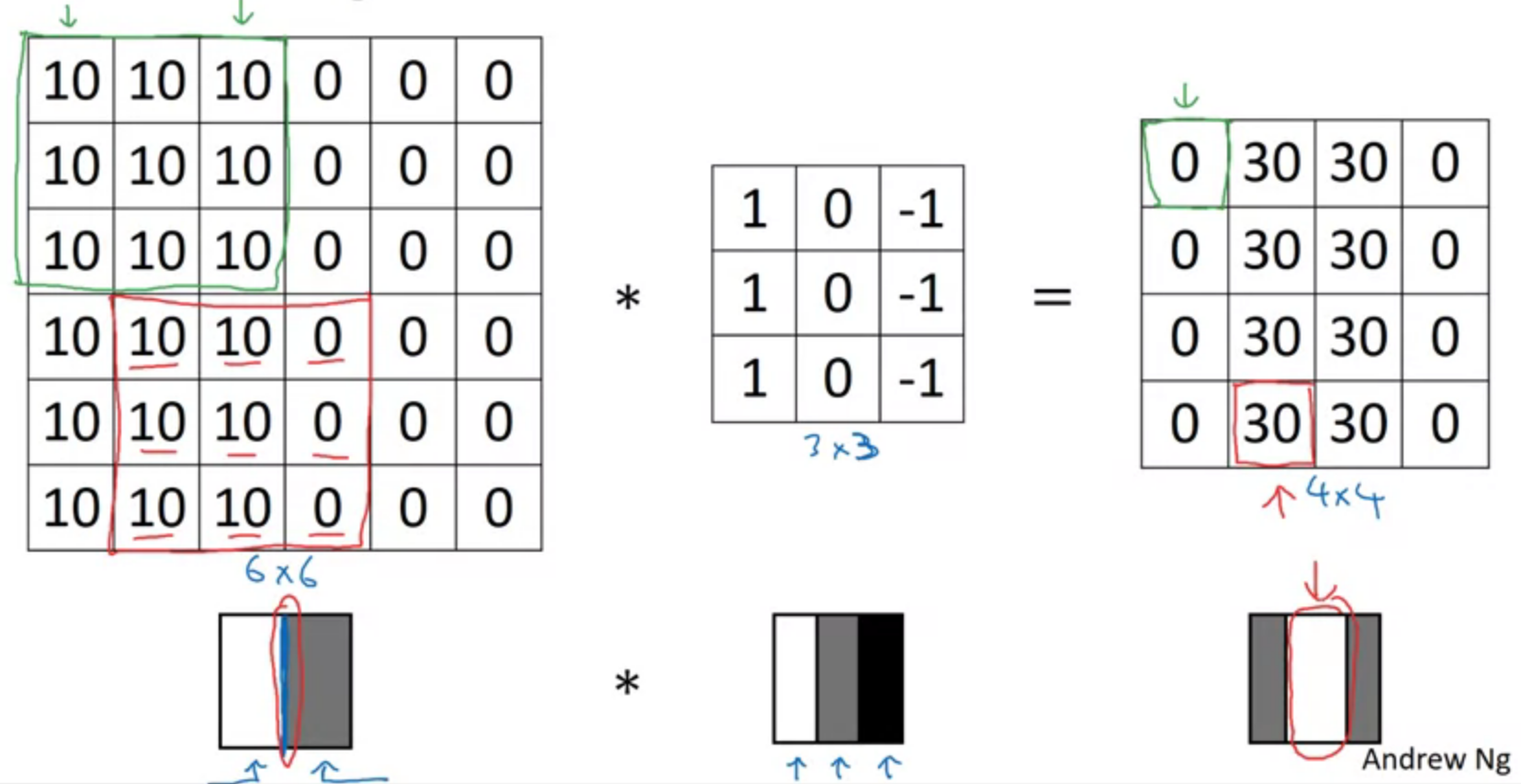Learning to detect edges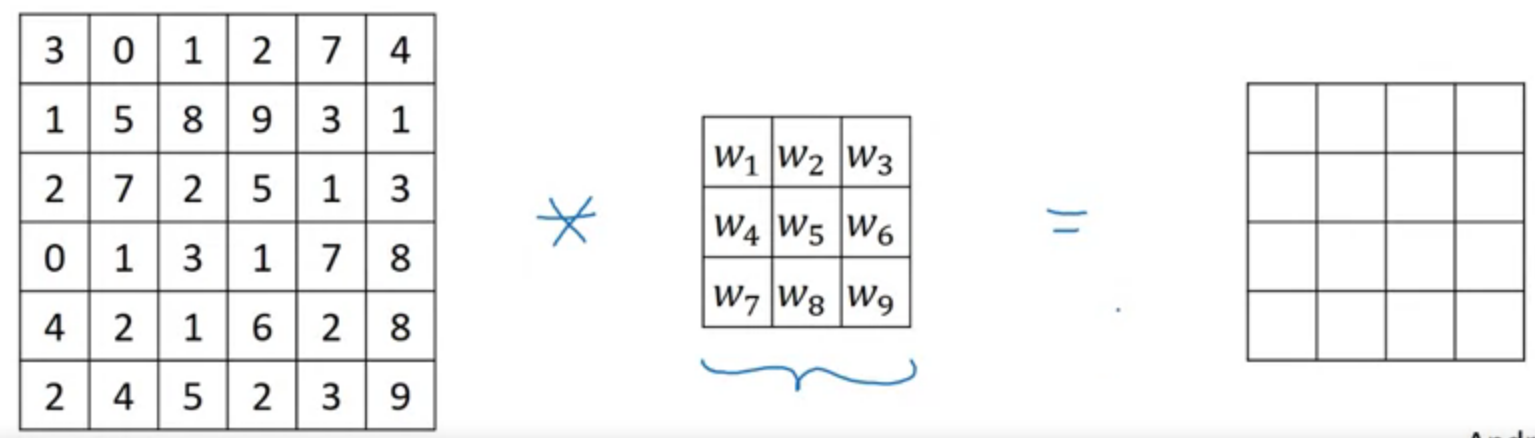Eg. horizontal, vertical, sobel, scharr filters.

image, $$n \times n$$
filter, $$f \times f$$

image after convolution, $$(n-f+1) \times (n-f+1)$$

## Valid convolution vs. same convolution

Valid convolution: no padding, $$n\times n * f\times f = (n - f + 1) * (n - f + 1)$$

Same convolution: pad so that output size is the same as the input size

$$n + 2p - f + 1 = n$$
means $$p = \frac{f - 1}{2}$$

$f$ is usually an odd number.

Same padding doesn’t change $n_H^{[l]}$ and $n_W^{[l]}$, while valid padding does. Pooling layers or valid padding can be used to downsize the height/width of the activation volumes. Sometimes they are helpful to build a deeper neural network.

## Benefits

• Use a Conv layer without necessarily shrinking the height and width of the volumes
• Keep more of the information at the border of an images. Without padding, very few values at the next layer would be affected by pixels as the edges of an image

## Problems

• shrink output
• throw away info from edge

## Strided convolutions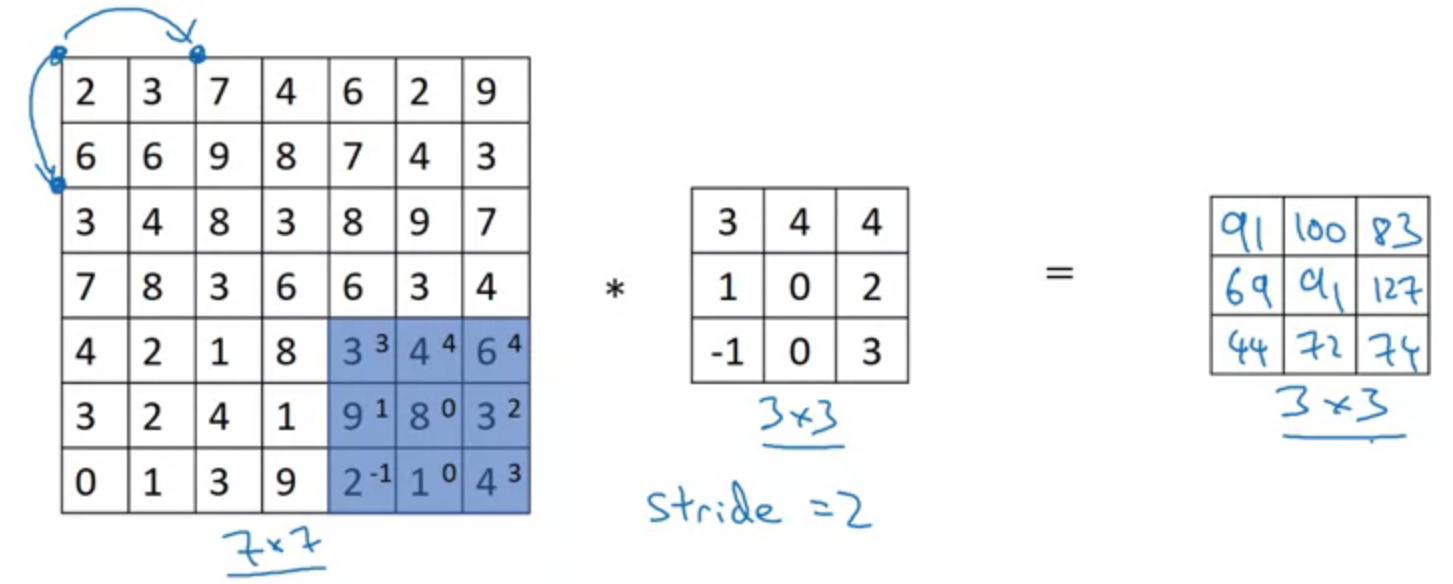$$[z] = floor(z)$$

Dimension

$$\left[ \frac{n + 2p - f}{s} + 1 \right] \times \left[ \frac{n + 2p - f}{s} + 1 \right]$$

# Convolution

## Technical note on cross-correlation vs convolution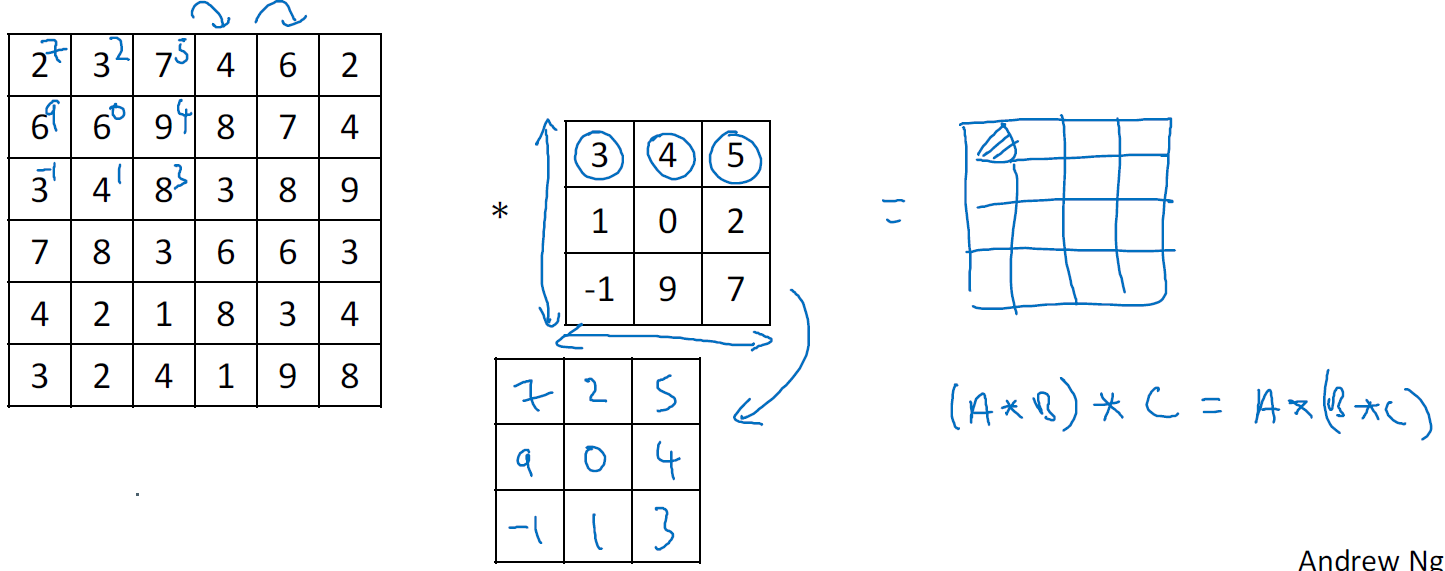And it follows

$$(A*B)*C = A*(B*C)$$

## Convolutions on RGB images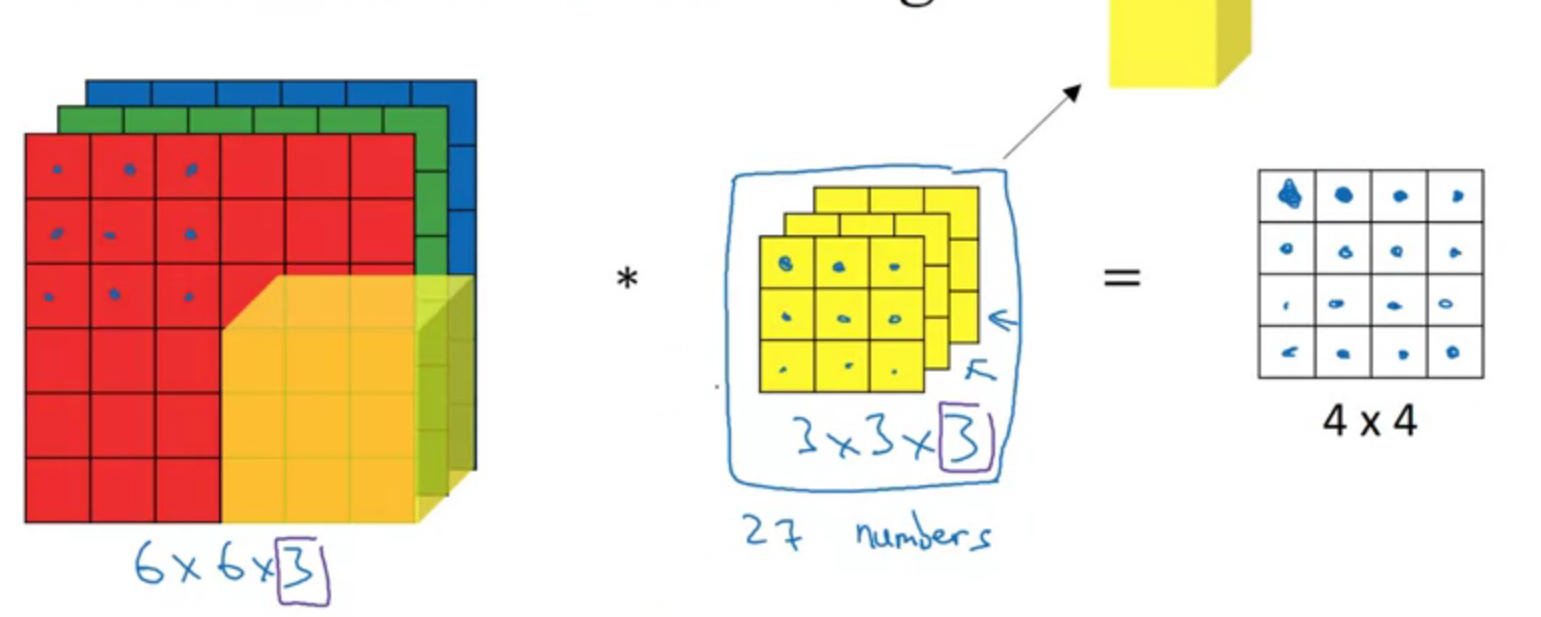Detect edges only in R channel

Detect edges only in R, G and B channel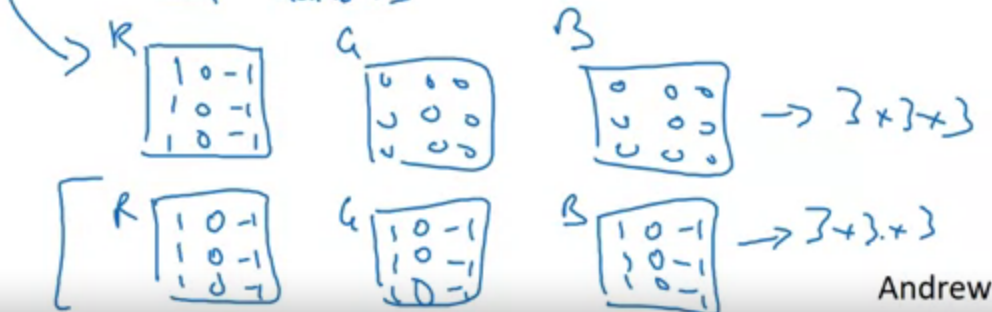## Multiple filters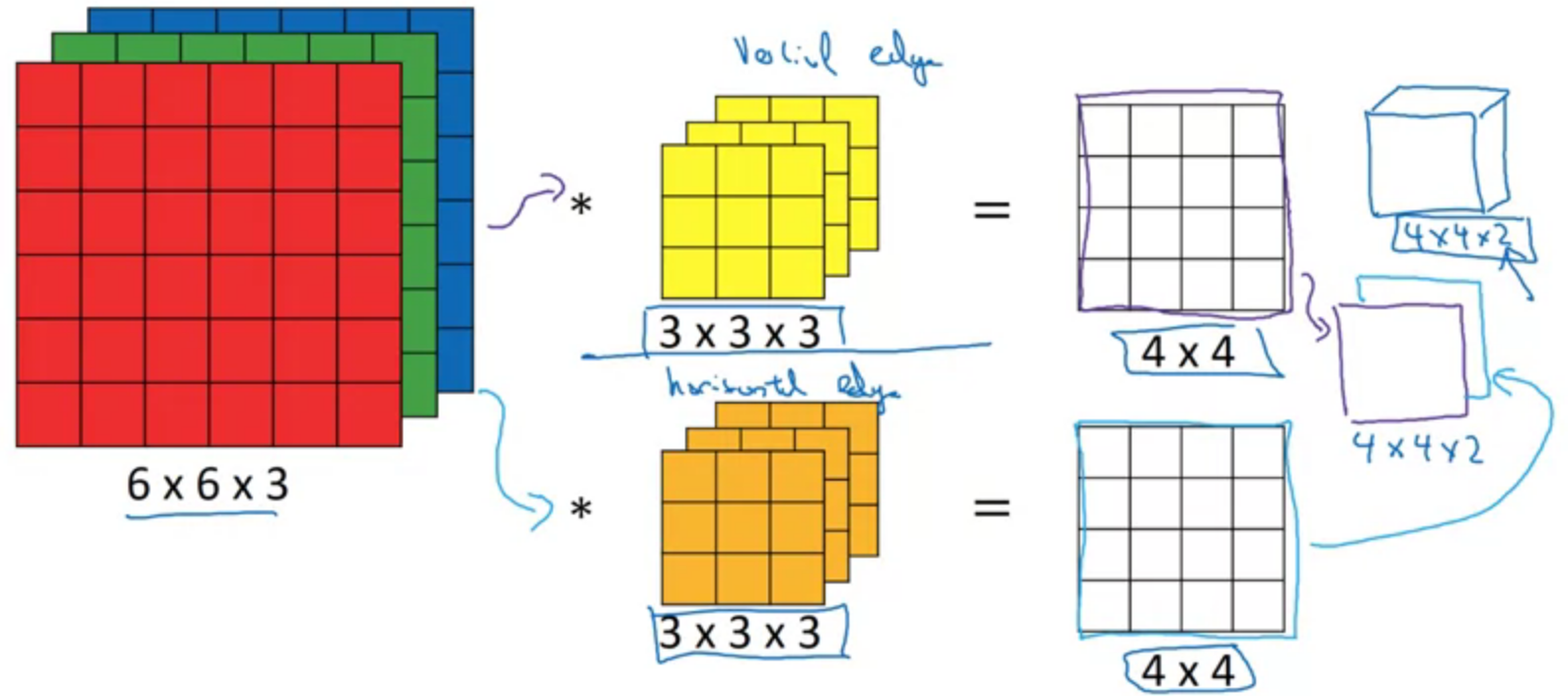$$(n \times n \times n_c) * (f \times f \times n_c) \to (n - f +1) \times (n - f + 1) \times n_c^{‘}$$

$n_c^{‘}$ is number of filters.

## One layer of a convolutional neural network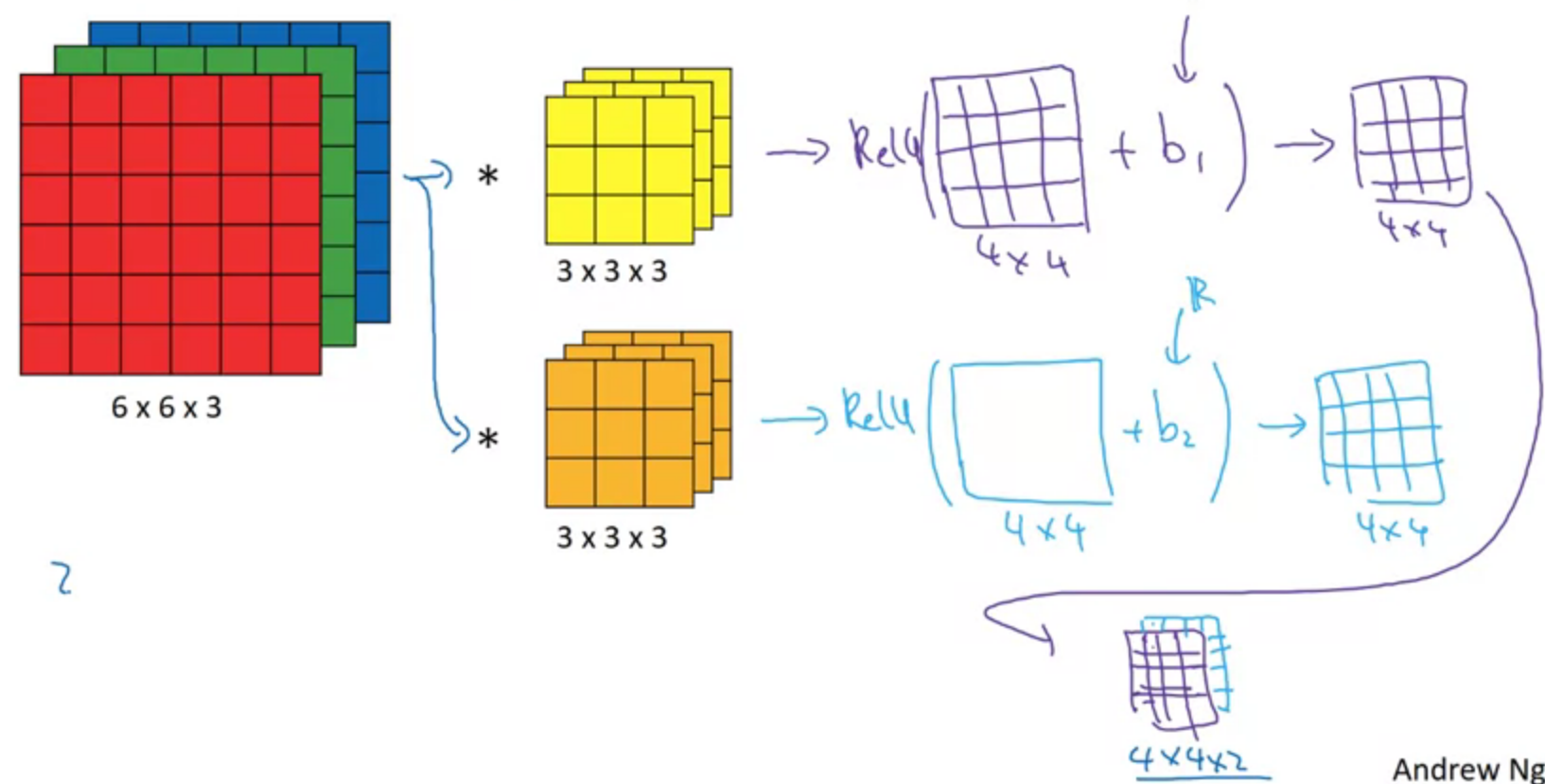$$z^{} = W^{} a^{} + b^{}$$

$$a^{} = g(z^{})$$

The parameters number is not relevant to each image size.

Q&A: If you have 10 filters that are 3 x 3 x 3 in one layer of a neural network, how many parameters does that layer have?

$$3 \times 3 \times 3 = 27$$

Plus the parameter of bias,

$$27 + 1 = 28$$

With 10 filters, it would be

$$28 \times 10 = 280$$

## Notation

$$f^{[l]} = \text{filter size}$$
$$p^{[l]} = \text{padding}$$
$$s^{[l]} = \text{stride}$$
$$n^{[l]}_c = \text{number of filters}$$

Each filter is: $$f^{[l]} \times f^{[l]} \times n^{[l - 1]}_c$$

Activations: $$a^{[l]} \to n^{[l]}_H \times n^{[l]}_W \times n^{[l]}_c$$
$$A^{[l]} \to m \times n^{[l]}_H \times n^{[l]}_W \times n^{[l]}_c$$

Weights: $$f^{[l]} \times f^{[l]} \times n^{[l - 1]}_c \times n^{[l]}_c$$
bias: $$(1, 1, 1, n^{[l]}_c)$$

Input: $$n^{[l - 1]}_H \times n^{[l - 1]}_W \times n^{[l - 1]}_c$$
Output: $$n^{[l]}_H \times n^{[l]}_W \times n^{[l]}_c$$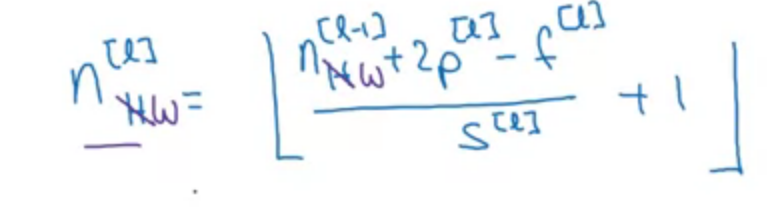## Example ConvNet

Height and width of images are gradually trending down while approaching in deeper layer. By contrast, the number of channels is increasing.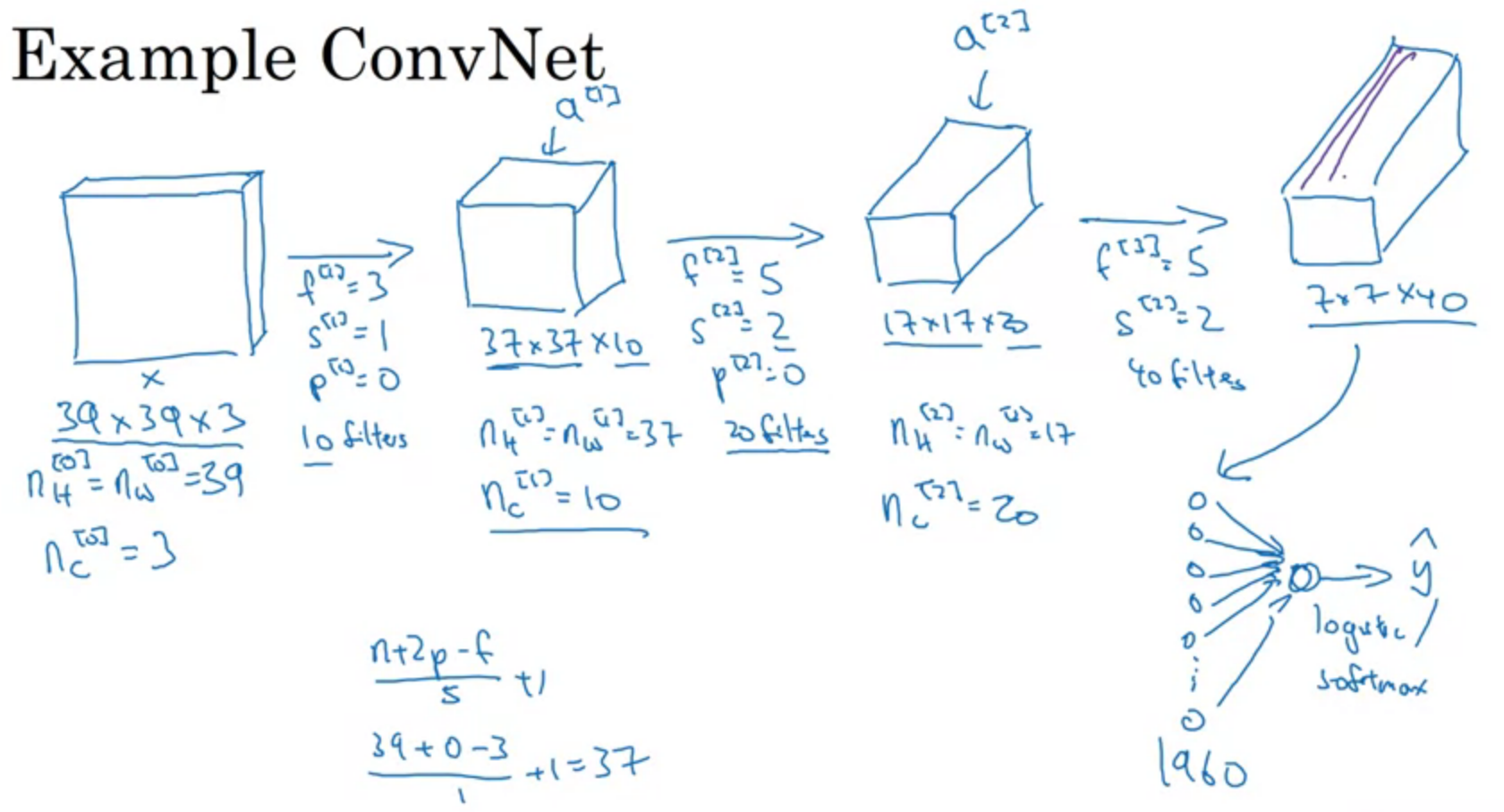Types of layer in a convolutional network

• convolution (conv)
• pooling (pool)
• fully connected (FC)

## Pooling layers

The function of pooling layers: To reduce variance, reduce computation complexity (as 2x2 max pooling/average pooling reduces 75% data) and extract low level features from neighbourhood.

Max pooling extracts the most important features like edges whereas, average pooling extracts features so smoothly.

Pooling stride each of the channel. 2D Pooling layer doesn’t not change the number of channels $n_c$.

Max pooling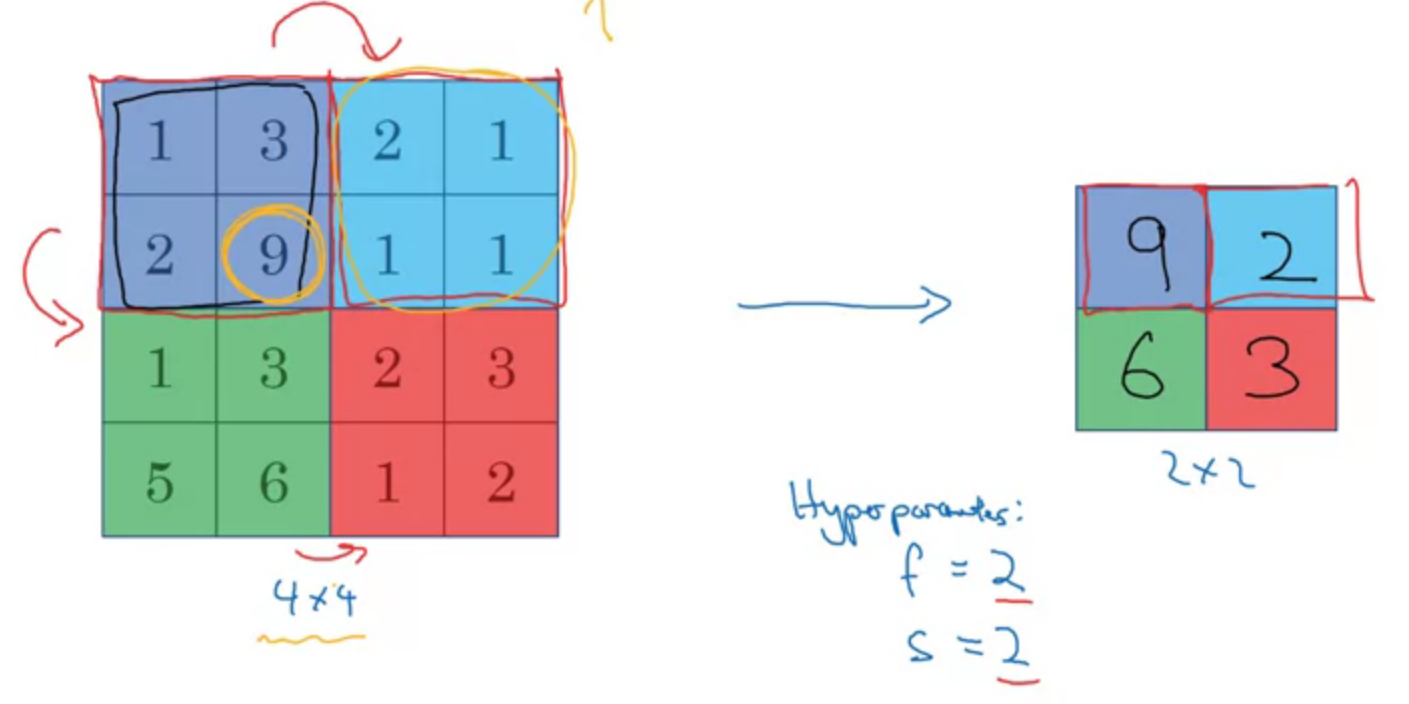Average pooling

Get the average number of pooling region on each step.

In Adaptive Pooling on the other hand, we specify the output size instead. And the stride and kernel-size are automatically selected to adapt to the needs. The following equations are used to calculate the value in the source code.

Stride = (input_size //output_size)
Kernel size = input_size - (output_size-1) * stride
Padding = 0

Hyperparameters

f: filter size
s: stride
max or average pooling
$$f = 2, s = 2$$
$$\left[ \frac{n_H - f}{s} + 1 \times \frac{n_W - f}{s} + 1 \right] \times n_c$$
No parameter to learn.

Example

LeNet-5

Conv-pool-conv-pool-FC-FC-FC-Softmax

eg.

Activation shape Activation size Parameters
Input (32,32,3) 3072 0
CONV1 (f=5, s=1) (28,28,8) 6272 608
POOL1 (14,14,8) 1568 0
CONV2 (f=5, s=1) (10,10,16) 1600 3216
POOL2 (5,5,16) 400 0
FC3 (120,1) 120 48120
FC4 (84,1) 84 10164
Softmax (10,1) 10 850

Calculus

For CONV, $$\text{No.} \text{parameters} = (f\times f + 1) \times n_c^{[l]}$$

For FC, $$\text{No.} \text{parameters} = (\text{activation size}_1 \times \text{activation size}_2) + \text{activation size}_2$$

## Why convolutional neural network?

Parameter sharing: a feature detector (such as a vertical edge detector) that’s useful in one part of the image is probably useful in another part of the image.
Sparsity of connections: In each layer, each output value depends only on a small number of inputs.
translation variance

## Putting it together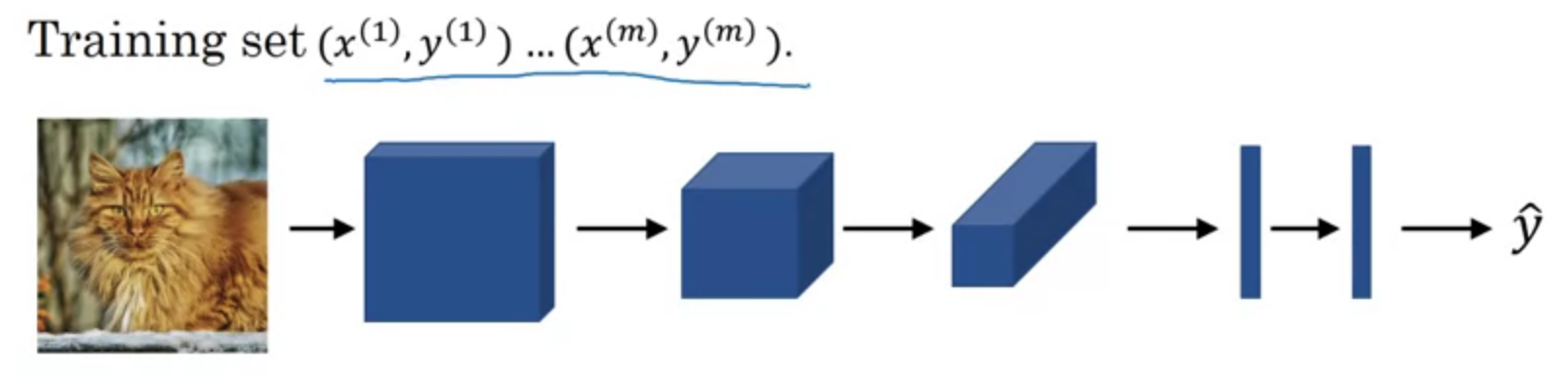$$Cost\ J = \frac{1}{m} \sum^m_{i=1} L(\hat{y}^{(i)}, y^{(i)})$$
Use gradient descent to optimize parameters to reduce $J$.

## Q&A

6.Suppose you have an input volume of dimension $n_H \times n_W \times n_C$. Which of the following statements you agree with? (Assume that “$1\times 1$ convolutional layer” below always uses a stride of 1 and no padding.)
You can use a 2D pooling layer to reduce $n_H$, $n_W$, and $n_C$.
-[x] You can use a 2D pooling layer to reduce $n_H$, $n_W$, but not $n_C$.
-[x] You can use a 1x1 convolutional layer to reduce $n_C$. but not $n_H$, $n_W$.
You can use a 1x1 convolutional layer to reduce $n_H$, $n_W$, and $n_C$.

# Reference

 Voulodimos, A., Doulamis, N., Doulamis, A. and Protopapadakis, E., 2018. Deep learning for computer vision: A brief review. Computational intelligence and neuroscience, 2018.

 Deeplearning.ai, Convolutional Neural Networks